# Damping with delta-time

Just a quick tip on how to convert usual damping code to something framerate-independent.

Most of us have probably, at some point, written code resembling this:

// Perform velocity damping
velocity -= velocity * 0.01f;


… or probably the more correct:

// Per-second damping coefficient
float const D = 10.0f;
// Damp velocity according to timestep
velocity -= velocity * D * delta_time;


Yet this is not fully framerate-independent; results are slightly different at 30fps and 60fps, and more importantly, spikes in the framerate cause lots of weird artifacts, causing developers to attempt to fix the situation by clamping delta_time, which is not ideal.

## The exponentiation method

Here is one way to fix it: assume that the code works correctly at 60 fps. This means that each frame, velocity is effectively multiplied by 1 - D / 60.

After one second, i.e. 60 frames, velocity has been multiplied by (1 - D / 60) ^ 60.

After two seconds, it has been multiplied by (1 - D / 60) ^ (60 * 2).

After N seconds, it has been multiplied by (1 - D / 60) ^ (60 * N).

So, there, we have a formula that tells us what happens after N seconds, and it’s a continuous function. We can therefore choose N as we like, and especially N = delta_time:

// Per-second damping coefficient
float const D = 10.0f;
// Damp velocity (framerate-independent)
velocity *= pow(1.f - D / 60.f, 60.f * delta_time);


Which can be conveniently rewritten as:

// Per-second damping coefficient
float const D = 10.0f;
// Exponentiation base for velocity damping
float const D2 = pow(1.f - D / 60.f, 60.f);
// Damp velocity (framerate-independent)
velocity *= pow(D2, delta_time);


# Quaternion from two vectors: the final version

I have published a first article about how to build a quaternion from two arbitrary direction vectors that will transform one of the directions into the other. That article was deliberately omitting the special case when the two vectors were facing away from each other, which required special treatment. So I wrote a second article explaining how to quickly pick an orthogonal vector.

Combining the results from the two articles should be straightforward. Here is the resulting, final function:

/* Build a unit quaternion representing the rotation
* from u to v. The input vectors need not be normalised. */
quat quat::fromtwovectors(vec3 u, vec3 v)
{
float norm_u_norm_v = sqrt(dot(u, u) * dot(v, v));
float real_part = norm_u_norm_v + dot(u, v);
vec3 w;
if (real_part < 1.e-6f * norm_u_norm_v)
{
/* If u and v are exactly opposite, rotate 180 degrees
* around an arbitrary orthogonal axis. Axis normalisation
* can happen later, when we normalise the quaternion. */
real_part = 0.0f;
w = abs(u.x) > abs(u.z) ? vec3(-u.y, u.x, 0.f)
: vec3(0.f, -u.z, u.y);
}
else
{
/* Otherwise, build quaternion the standard way. */
w = cross(u, v);
}
return normalize(quat(real_part, w.x, w.y, w.z));
}


If you know you are exclusively dealing with unit vectors, you can replace all occurrences of norm_u_norm_v with the value 1.0f in order to avoid a useless square root.

# On picking an orthogonal vector (and combing coconuts)

Consider the following problem:

Given a non-zero vector v in 3D space, find a non-zero vector w orthogonal to v (i.e. such that v.w = 0).

At first sight, this seems easy. For every possible v there are an infinite number of orthogonal vectors lying in a plane:We just need to pick one. But it’s not as trivial as it may seem.

## First attempts

The following vector satisfies v.w1 = 0:Unfortunately that w1 vector can be zero, for instance when v = [0 0 1]. The original problem requires a non-zero vector.

Let’s try another one: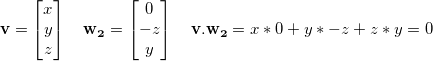Again, it works most of the time, except in some cases, for instance v = [1 0 0].

How about adding them together? Certainly w1 and w2 cannot be both zero at the same time!Alas, this still doesn’t work all the time: it fails with v = ![1 0 1].

Should we try harder? Isn’t there a linear combination of these that will work all the time?

Well, no. There isn’t.

## You can’t comb a coconut

Sadly, the hairy ball theorem, a consequence of Brouwer’s fixed-point theorem, states that any continuous function f that maps the set of unit vectors to orthogonal vectors takes the value zero somewhere.

Whichever way you look at the sphere of unit vectors, a continuous tangent field will always have a zero point:Most of the maths operations we usually do (adding, multiplying, taking square roots, etc.) are continuous. Fortunately, we are not limited to them: we can use a simple if() construct to create a discontinuity that will save us.

## A very good implementation

Here is my personal implementation of the orthogonal vector problem. It is very good. It has excellent numerical stability. It only does one test. It is short. It is beautiful. I really hope you consider using it.

/* Always works if the input is non-zero.
* Doesn’t require the input to be normalised.
* Doesn’t normalise the output. */
vec3 orthogonal(vec3 v)
{
return abs(v.x) > abs(v.z) ? vec3(-v.y, v.x, 0.0)
: vec3(0.0, -v.z, v.y);
}


Many implementations, such as Ogre3D’s, have additional tests or operations to perform this task, and use epsilons and normalised vectors to avoid singularities.

But our only test is actually enough: if |x|>|z|, it means the largest component in absolute value in v is either x or y. So we build a vector using x and y. Otherwise, the largest component in absolute value is either y or z. So we build a vector using y and z. That way, we ensure that the length of our returned vector never, ever comes near zero.

Whether some given code will cause inefficient branching is often unclear. In our case, it is very likely that the ternary operator will actually be branchless, with some help of the compiler and the hardware.

That said, how about we try to get rid of the ternary operator, just for fun?

## Going branchless for fun

Let’s see. We had these two candidate vectors, w1 and w2, which worked almost always except when specific values of v caused them to be zero. And whatever the constant k we may pick, a vector of the form w1 + k w2 will eventually take the value zero for some value of v, too, because of the hairy ball theorem.

Now here comes the trick. Instead of using a constant k, we use a function f(x,y,z). This is what our w vector looks like: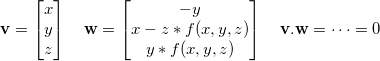From now I shall assume that v is a unit vector.

What can cause w to be zero, then?

One necessary condition is y = 0. When y ≠ 0 we can chose anything we want for f(x,y,z), it’ll always give us a good orthogonal vector. This restricts the problem to the y = 0 circle, giving us the useful equality x² + z² = 1.

The other condition is x = z*f(x,y,z). So if we manage to build a function f such that f(x,y,z) never equals x/z, we win.

Using x² + z² = 1 we can plot all the possible values for x/z as a function of x. It will show us, for a given x, the values that f cannot take:The almost vertical slopes you see go to infinity upwards and downwards. As expected, this prevents us from using a continuous function: it would obviously cross one of these walls at some point.

Well, let’s try a non-continuous function, then. What are our options?

• fmod
• floor, ceil, round
• fract

Here is one that I came up with and which works reasonably well: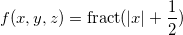Look how it nicely avoids x/z:And finally, our resulting branchless code:

/* Requires the input to be normalised.
* Doesn’t normalise the output. */
vec3 orthogonal(vec3 v)
{
float k = fract(abs(v.x) + 0.5);
return vec3(-v.y, v.x - k * v.z, k * v.y);
}


I find it highly unlikely that this second version will perform better than the branching one. However, I haven’t put much thought into it and maybe someone will come up with a much better solution using the ideas presented here. Have fun!

# Beautiful maths simplification: quaternion from two vectors

In this article I would like to explain the thought process behind the derivation of a widely used formula: finding a quaternion representing the rotation between two 3D vectors. Nothing really new, but hopefully a few ideas could be reused at other times.

Note: the routine presented here is incomplete on purpose. For a version that can be used in production code, see the next article instead, Quaternion from two vectors: the final version.

## Naive method

A rotation is best visualised using a rotation axis and an angle. Except in degenerate cases, the rotation axis can be obtained by computing the cross product of the two original vectors:Then the angle can be obtained using the properties of the cross product and/or the dot product:Since θ is always between 0 and π, we only care about the dot product. This gives us some obvious code to create the quaternion (omitting corner cases such as θ = 0 for clarity):

quat quat::fromtwovectors(vec3 u, vec3 v)
{
float cos_theta = dot(normalize(u), normalize(v));
float angle = acos(cos_theta);
vec3 w = normalize(cross(u, v));
return quat::fromaxisangle(angle, w);
}


This is naive but it works. Googling for “quaternion from two vectors” shows this forum post and this SO question where this method or a variation thereof is suggested.

## Looking under the hood

Let’s have a look at what happens when building the quaternion from an axis and an angle: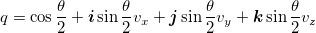This means the code for quat::fromaxisangle would look somewhat like this:

quat quat::fromaxisangle(float angle, vec3 axis)
{
float half_sin = sin(0.5f * angle);
float half_cos = cos(0.5f * angle);
return quat(half_cos,
half_sin * axis.x,
half_sin * axis.y,
half_sin * axis.z);
}


## Avoiding trigonometry

If you read Iñigo Quilez’s recent article about avoiding trigonometry you’ll have probably frowned at the fact that we computed θ from cos(θ), then computed sin(θ/2) and cos(θ/2).

Indeed, it happens that there is a much simpler way to do it; the half-angle formulas from precalculus tell us the following: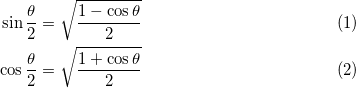This allows us to simplify our quaternion creation code:

quat quat::fromtwovectors(vec3 u, vec3 v)
{
float cos_theta = dot(normalize(u), normalize(v));
float half_cos = sqrt(0.5f * (1.f + cos_theta));
float half_sin = sqrt(0.5f * (1.f - cos_theta));
vec3 w = normalize(cross(u, v));
return quat(half_cos,
half_sin * w.x,
half_sin * w.y,
half_sin * w.z);
}


This is pretty nice. By using well known trigonometry formulas, we got rid of all trigonometry function calls!

## Avoiding square roots

It happens that we can do slightly better. Note that we normalize three vectors: u, v and cross(u, v). That’s three square roots. The thing is, we already know the norm of w through this formula: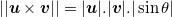And we know sin(θ) from precalculus again: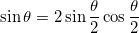Also, using the fact that sqrt(a)sqrt(b) = sqrt(ab) lets us perform one less square root.

We can therefore come up with the following performance improvement:

quat quat::fromtwovectors(vec3 u, vec3 v)
{
float norm_u_norm_v = sqrt(sqlength(u) * sqlength(v));
float cos_theta = dot(u, v) / norm_u_norm_v;
float half_cos = sqrt(0.5f * (1.f + cos_theta));
float half_sin = sqrt(0.5f * (1.f - cos_theta));
vec3 w = cross(u, v) / (norm_u_norm_v * 2.f * half_sin * half_cos);
return quat(half_cos,
half_sin * w.x,
half_sin * w.y,
half_sin * w.z);
}


Oh wait! We divide by sin(θ/2) to compute w, then we multiply by sin(θ/2) again. This means we don’t even need that variable, and we can simplify even further:

quat quat::fromtwovectors(vec3 u, vec3 v)
{
float norm_u_norm_v = sqrt(sqlength(u) * sqlength(v));
float cos_theta = dot(u, v) / norm_u_norm_v;
float half_cos = sqrt(0.5f * (1.f + cos_theta));
vec3 w = cross(u, v) / (norm_u_norm_v * 2.f * half_cos);
return quat(half_cos, w.x, w.y, w.z);
}


This is more or less the code used by the Ogre3D engine in OgreVector3.h, except they perform an additional normalisation step on the final result. This is mathematically useless, but due to numerical stability issues, it is probably safe to do so nonetheless.

This final normalisation step is actually an opportunity to simplify the code even further.

## Improving on Ogre3D

We are down to two square roots and four divisions, plus quite a few mul/adds. Depending on the platform that we are running on, it is possible to simplify even further and improve performance. For instance, on many SIMD architectures, normalising a quaternion can be very fast.

This is the code we get if we multiply every component of the quaternion by 2.f * half_cos and let normalize() do the rest of the job:

quat quat::fromtwovectors(vec3 u, vec3 v)
{
float norm_u_norm_v = sqrt(sqlength(u) * sqlength(v));
float cos_theta = dot(u, v) / norm_u_norm_v;
float half_cos = sqrt(0.5f * (1.f + cos_theta));
vec3 w = cross(u, v) / norm_u_norm_v;
return normalize(quat(2.f * half_cos * half_cos, w.x, w.y, w.z));
}


Now half_cos only appears in its squared form, and since it comes from a square root, we can simply omit that square root:

quat quat::fromtwovectors(vec3 u, vec3 v)
{
float norm_u_norm_v = sqrt(sqlength(u) * sqlength(v));
float cos_theta = dot(u, v) / norm_u_norm_v;
vec3 w = cross(u, v) / norm_u_norm_v;
return normalize(quat(1.f + cos_theta, w.x, w.y, w.z));
}


And using the same reasoning we can multiply every quaternion component by norm_u_norm_v:

quat quat::fromtwovectors(vec3 u, vec3 v)
{
float norm_u_norm_v = sqrt(sqlength(u) * sqlength(v));
vec3 w = cross(u, v);
quat q = quat(norm_u_norm_v + dot(u, v), w.x, w.y, w.z);
return normalize(q);
}


We are still doing two square roots and four divisions, some of which are hidden in normalize(), but the code is considerably shorter now.

## Final formIf u and v can be enforced to be unit vectors, norm_u_norm_v can be omitted and simply replaced with 1.0f:

quat quat::fromtwovectors(vec3 u, vec3 v)
{
vec3 w = cross(u, v);
quat q = quat(1.f + dot(u, v), w.x, w.y, w.z);
return normalize(q);
}


Isn’t it beautiful, considering the sin(), cos() and acos() ridden mess we started with?

This algorithm can be found all over the Internet, but I do not know who first came up with it. Also, a lot of 3D engines (both publicly available and slightly more private) could benefit from it.

## Update (06/01/2014)

In the comments below, Michael Norel provides the following improvement to the non-unit version. Since the values d = dot(u, v) and w = cross(u, v) are computed no matter what, the value sqlength(u) * sqlength(v) could be computed in a different way, *i.e.* d * d + sqlength(w). The following code does at least three multiplications less:

quat quat::fromtwovectors(vec3 u, vec3 v)
{
vec3 w = cross(u, v);
quat q = quat(dot(u, v), w.x, w.y, w.z);
q.w += length(q);
return normalize(q);
}


Also, Marc B. Reynolds notes that, in the unit version, the final normalisation factor is sqrt((1 + dot(u, v))² + sqlength(cross(u, v))) which reduces to sqrt(2 + 2 dot(u, v)) thanks to the sin² + cos² identity. It leads to the following possibly improved version:

quat quat::fromtwovectors(vec3 u, vec3 v)
{
float m = sqrt(2.f + 2.f * dot(u, v));
vec3 w = (1.f / m) * cross(u, v);
return quat(0.5f * m, w.x, w.y, w.z);
}


# Maths trick: doing fewer comparisons

Note: this is not an optimisation. It is just one more tool you should have in your toolbox when looking for optimisations. It may be useful.

This is the trick: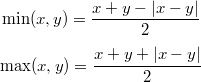You can check for yourself that it is always true: when x > y, |x - y| is the same as x - y, etc.

What good is it for? There is often an implicit comparison in min or max. It might be interesting to replace it with a call to the branchless fabs.

## Example usage

Consider the following code:

float a, b, c, d;
/* ... */
return (a > b) && (c > d);


That kind of code is often used eg. in collision checks, where a lot of tests can be done. This code does two comparisons. On some architectures, this means two branches. Not always something you want.

The test condition is equivalent to:

(a - b > 0) && (c - d > 0)


Now when are two given numbers both positive? That is if and only if the smallest is positive:

min(a - b, c - d) > 0


We may now use our trick:

(a - b) + (c - d) - |(a - b) - (c + d)| > 0


And so the code could be rewritten as such:

float a, b, c, d;
/* ... */
return (a - b) + (c - d) > fabsf((a - b) - (c - d));


We basically replaced the additional test with a call to fabsf and some additions/subtractions. It may be possible to reorganise the input data so that this second version performs better.

# Announce: LolRemez 0.2 released

A new version of our high precision polynomial approximation solver, LolRemez 0.2, is available.

The changes, taking into account all the feedback users provided, are as follows:

• significant performance and accuracy improvements thanks to various bugfixes and a better extrema finder for the error function.
• user can now define accuracy of the final result.
• exp, sin, cos and tan are now about 20% faster.
• multiplying a real number by an integer power of two is now a virtually free operation.
• fixed a rounding bug in the real number printing routine.

You can visit the software homepage to download LolRemez and, more importantly, the comprehensive documentation featuring a step-by-step tutorial.

# Announce: LolRemez 0.1 released

In my previous article about the Remez exchange algorithm I said I was working on a Remez exchange toolkit for everyone to play with. Though it’s far from being full-featured, I already use it and I believe it is already extremely useful. So I decided to release LolRemez 0.1 to the masses.

You can visit the software homepage to download LolRemez and, more importantly, the comprehensive documentation featuring a step-by-step tutorial.

# Better function approximations: Taylor vs. Remez

You may have once crossed this particular piece of magic: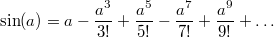The right part is the Taylor series of sin around 0. It converges very quickly to the actual value of sin(a). This allows a computer to compute the sine of a number with arbitrary precision.

And when I say it’s magic, it’s because it is! Some functions, called the entire functions, can be computed everywhere using one single formula! Other functions may require a different formula for different intervals; they are the analytic functions, a superset of the entire functions. In general, Taylor series are an extremely powerful tool to compute the value of a given function with very high accuracy, because for several common functions such as sin, tan or exp the terms of the series are easy to compute and, when implemented on a computer, can even be stored in a table at compile time.

## Approximating sin with Taylor series

This is how one would approximate sin using 7 terms of its Taylor series on the [-π/2, π/2] interval. The more terms, the better the precision, but we’ll stop at 7 for now:

static double taylorsin(double x)
{
static const
double a0 =  1.0,
a1 = -1.666666666666666666666666666666e-1,  /* -1/3! */
a2 =  8.333333333333333333333333333333e-3,  /*  1/5! */
a3 = -1.984126984126984126984126984126e-4,  /* -1/7! */
a4 =  2.755731922398589065255731922398e-6,  /*  1/9! */
a5 = -2.505210838544171877505210838544e-8,  /* -1/11! */
a6 =  1.605904383682161459939237717015e-10; /*  1/13! */
double x2 = x * x;
return x * (a0 + x2 * (a1 + x2 * (a2 + x2
* (a3 + x2 * (a4 + x2 * (a5 + x2 * a6))))));
}


And you may think…“Oh wow that is awesome! So simple for such a difficult function. Also, since I read your masterpiece about polynomial evaluation I know how to improve that function so that it is very fast!”

Well, actually, no.If you are approximating a function over an interval using its Taylor series then either you or the person who taught you is a fucking idiot because a Taylor series approximates a function near a fucking point, not over a fucking interval, and if you don’t understand why it’s important then please read on because that shit is gonna blow your mind.

## Error measurement

Let’s have a look at how much error our approximation introduces. The formula for the absolute error is simple: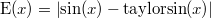And this is how it looks like over our interval:You can see that the error skyrockets near the edges of the [-π/2, π/2] interval.“Well the usual way to fix this is to split the interval in two or more parts, and use a different Taylor series for each interval.”

Oh, really? Well, let’s see the error on [-π/4, π/4] instead:I see no difference! The error is indeed smaller, but again, it becomes extremely large at the edges of the interval. And before you start suggesting reducing the interval even more, here is the error on [-π/8, π/8] now:I hope this makes it clear that:

• the further from the centre of the interval, the larger the error
• the error distribution is very unbalanced
• the maximum error on [-π/2, π/2] is about 6.63e-10

And now I am going to show you why that maximum error value is pathetic.

## A better approximation

Consider this new function:

static double minimaxsin(double x)
{
static const
double a0 =  1.0,
a1 = -1.666666666640169148537065260055e-1,
a2 =  8.333333316490113523036717102793e-3,
a3 = -1.984126600659171392655484413285e-4,
a4 =  2.755690114917374804474016589137e-6,
a5 = -2.502845227292692953118686710787e-8,
a6 =  1.538730635926417598443354215485e-10;
double x2 = x * x;
return x * (a0 + x2 * (a1 + x2 * (a2 + x2
* (a3 + x2 * (a4 + x2 * (a5 + x2 * a6))))));
}


It doesn’t look very different, right? Right. The values a0 to a6 are slightly different, but the rest of the code is strictly the same.

Yet what a difference it makes! Look at this error curve:That new function makes it obvious that:

• the error distribution is better spread over the interval
• the maximum error on [-π/2, π/2] is about 4.96e-14

Check that last figure again. The new maximum error isn’t 10% better, or maybe twice as good. It is more than ten thousand times smaller!!

## The minimax polynomial

The above coefficients describe a minimax polynomial: that is, the polynomial that minimises a given error when approximating a given function. I will not go into the mathematical details, but just remember this: if the function is sufficiently well-suited (as sin, tan, exp etc. are), then the minimax polynomial can be found.

The problem? It’s hard to find. The most popular algorithm to find it is the Remez exchange algorithm, and few people really seem to understand how it works (or there would be a lot less Taylor series). I am not going to explain it right now. Usually you need professional math tools such as Maple or Mathematica if you want to compute a minimax polynomial. The Boost library is a notable exception, though.

But you saw the results, so stop using Taylor series. Spending some time finding the minimax polynomial is definitely worth it. This is why I am working on a Remez framework that I will make public and free for everyone to use, modify and do what the fuck they want. In the meantime, if you have functions to numerically approximate, or Taylor-based implementations that you would like to improve, let me know in the comments! This will be great use cases for me.